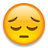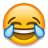# IDFC Select Credit Card 10x Reward Program

55°
bdatta1981

Recently I paid school fees of Rs 27,360 using my IDFC First Select Credi Card to utilize their 10x reward program for spending of >20K.

The credit card bill got generated 2 days back and I am surprised to see the points awarded for the >20K spend!Their website mentions 1X = 1 Reward point per ₹100 spent | 1 Reward point = ₹0.25. So 6X reward points should be 6 point per Rs 100 spent and 10X reward should be 10 reward points per Rs 100 spent. Using that calculation, Rs 27360 should calculate to 2736 points. Even if we consider that 10x applies to amount only >20K, so Rs 7360 should calculate to 736 points.

So either I was expecting points earned as 2736 or (1642 + 736) = 2378. In actual they have credited only 1936 points!

Does anyone know how is their reward points calculated?

Expiring In 1 day
6 Dimers
• Sort By

1200+736 so you got 1936. They are correct. 10x is applicable only on the incremental spends above 20k not the total spends

They calculate 6x of the whole amount and then 4x above 20k. So the points you have received are more or less correct.

For 20000 it is 6x
1200 pts = 300rs

For 7360 = 10x
736 = 184 rs

prem4998 wrote:

1200+736 so you got 1936. They are correct. 10x is applicable only on the incremental spends above 20k not the total spends

Thanks, buddy. Makes sense to me now.

I was assuming that they give 10x reward on the total and not on the incremental spendLooks like 10x reward program is a waste. 1936 points calculates to Rs 484.
Had I used my Axis ACE credit card, I would have got 2% cashback on 27,360 = Rs 547.20 much better than the IDFC.

Expand
bdatta1981 wrote:

Thanks, buddy. Makes sense to me now.

I was assuming that they give 10x reward on the total and not on the incremental spendLooks like 10x reward program is a waste. 1936 points calculates to Rs 484.
Had I used my Axis ACE credit card, I would have got 2% cashback on 27,360 = Rs 547.20 much better than the IDFC.

On top of that they r gonna start charging redemption fee soon(around October i think)Expand
bdatta1981 wrote:

Thanks, buddy. Makes sense to me now.

I was assuming that they give 10x reward on the total and not on the incremental spendLooks like 10x reward program is a waste. 1936 points calculates to Rs 484.
Had I used my Axis ACE credit card, I would have got 2% cashback on 27,360 = Rs 547.20 much better than the IDFC.

Yes, Ace is better in this case. But if your total spends are 40k+, then final valueback from IDFC would be more than 2% from Ace

Expand
MrMonkey wrote:

Yes, Ace is better in this case. But if your total spends are 40k+, then final valueback from IDFC would be more than 2% from Ace

Interesting. Thanks for sharing this useful tip!

Expand
MrMonkey wrote:

Yes, Ace is better in this case. But if your total spends are 40k+, then final valueback from IDFC would be more than 2% from Ace

After the recent devaluation of idfc reward system,ace wins